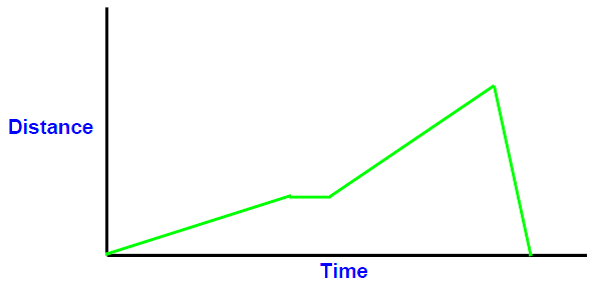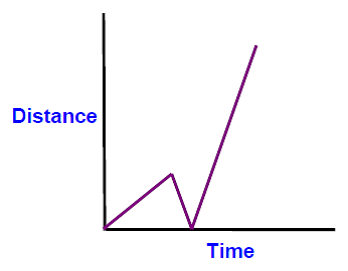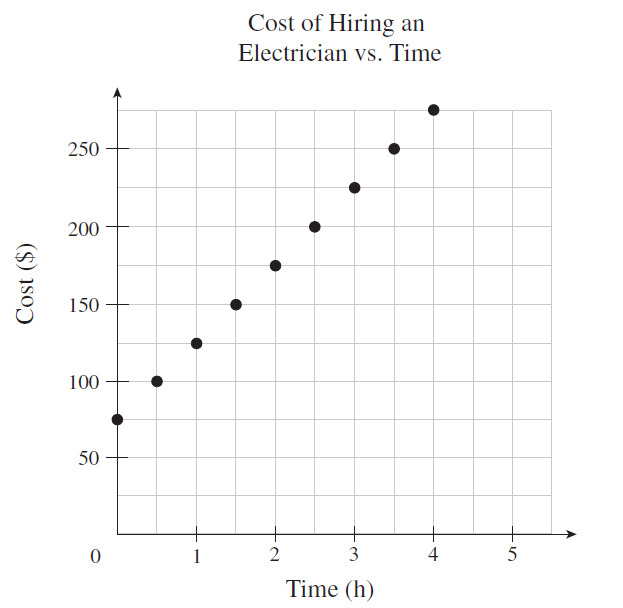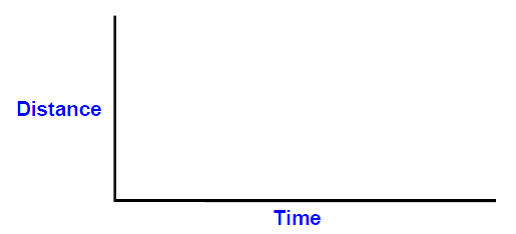# Understand relations between x- and y-intercepts

### Understand relations between x- and y-intercepts

The relations between x-and y-intercepts can go beyond mathematics. It can represent the various kinds of real-life scenarios. For example, time and distance and money related problems.

#### Lessons

• 1.
Describe a possible scenario for each graph
a)b)c)
What is the cost of hiring an electrician for 8 hours?• 2.
Sketch a graph for each scenario
a)
A runner is competing in the 2000 meter race from start to finish line